# 0708-1300/Homework Assignment 5

Announcements go here
In Preparation

The information below is preliminary and cannot be trusted! (v)

## Contents

Read sections 1-3 of chapter V of Bredon's book three times:

• First time as if you were reading a novel - quickly and without too much attention to detail, just to learn what the main keywords and concepts and goals are.
• Second time like you were studying for an exam on the subject - slowly and not skipping anything, verifying every little detail.
• And then a third time, again at a quicker pace, to remind yourself of the bigger picture all those little details are there to paint.

Also, do the same with your own class notes - much of what we do for this part of the class is not in the textbook!

## Doing

Solve all of the following problems, but submit only your solutions of problems *,* and *:

Problem 1. Let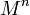$M^n$ be a manifold. Show that the following definitions for the orientability of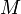$M$ are equivalent:

1. There exists a nowhere vanishing$n$-form on$M$.
2. There exists an atlas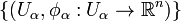$\{(U_\alpha,\phi_\alpha:U_\alpha\to{\mathbb R}^n)\}$ for$M$, so that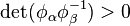$\det(\phi_\alpha\phi^{-1}_\beta)>0$ wherever that makes sense.

Problem 2. Show that the tangent space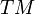$TM$ of any manifold$M$ is orientable.

Problem 3.

1. Show that if$M$ and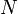$N$ are orientable then so is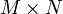$M\times N$.
2. Show that if$M$ and$M\times N$ are orientable then so is$N$.

Problem 4. Show that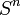$S^n$ is always orientable.

Problem 5. Recall that a form is called closed if it is in the kernel of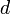$d$ and exact if it is in the image of$d$. Show that every exact form is closed.

Problem 6. Let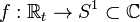$f:{\mathbb R}_t\to S^1\subset{\mathbb C}$ be given by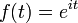$f(t)=e^{it}$.

1. Show that there exists a unique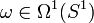$\omega\in\Omega^1(S^1)$ such that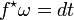$f^\star\omega=dt$.
2. Show that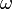$\omega$ is closed but not exact.

Problem 7. Show, directly from the definitions, that every closed 1-form on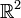${\mathbb R}^2$ is exact.

Problem 8. Compute the integral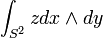$\int_{S^2}zdx\wedge dy$ twice:

1. Using Stokes' theorem.
2. Directly from the definition, by using a one- or two-chart atlas for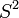$S^2$.

(Repeat 1 and 2 until they stop giving different answers).

Problem 9. Show that the form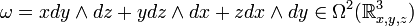$\omega=xdy\wedge dz+ydz\wedge dx+zdx\wedge dy\in\Omega^2({\mathbb R}^3_{x,y,z})$ is invariant under rigid orientation-preserving rotations of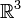${\mathbb R}^3$. That is, if$A$ is such a rotation matrix (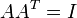$AA^T=I$ and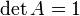$\det A=1$) considered also as a linear transformation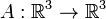$A:{\mathbb R}^3\to{\mathbb R}^3$, then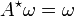$A^\star\omega=\omega$.

## Due Date

This assignment is due in class on Thursday December 6, 2007.